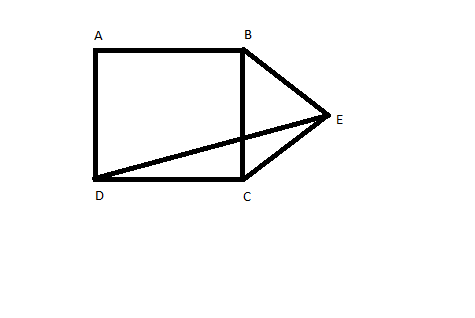Question 11

# If ABCD is a square and BCE is an equilateral triangle, what is the measure of ∠DEC?Solution

According to given diagram, as triangle BCE is equilateral CE=BE=DC
Hence angle CDE = angle CED

So angle DEC = $$\frac{180-150}{2} = 15$$